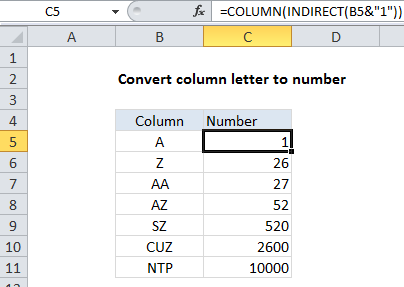## Excel Office

Excel How Tos, Tutorials, Tips & Tricks, Shortcuts

# Convert column letter to number in Excel

To convert a column letter to an regular number (e.g. 1, 10, 26, etc.) you can use a formula based on the INDIRECT and COLUMN functions. See example below:

## Formula

`=COLUMN(INDIRECT(letter&"1"))`## Explanation

In the example shown, the formula in C5 is:

`=COLUMN(INDIRECT(B5&"1"))`

### How this formula works

The first step is to construct a standard “A1” style reference using the column letter, by adding a “1” with concatenation:

`B5&"1"`

This results in a text string like “A1” which is passed into the INDIRECT function.

Also See:   How to get first column number in range in Excel

Next, the INDIRECT function transforms the text into a proper Excel reference and hands the result off to the COLUMN function.

Finally, the COLUMN function evaluates the reference and returns the column number for the reference.